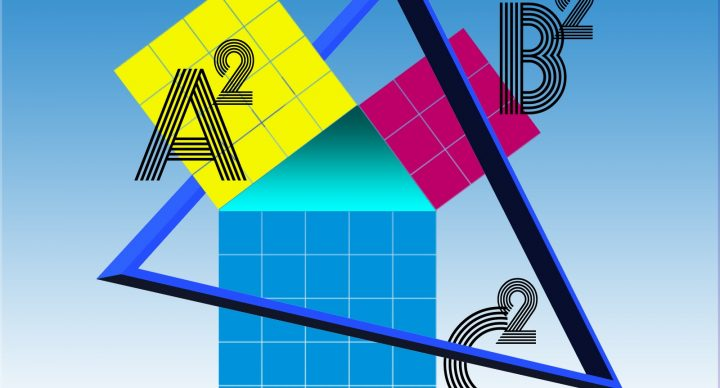### Course Overview

In the Acellus Grade 6 Math course, students study the mathematical concepts that will help them as they transition through middle school and prepare for Pre-Algebra.  Mr. Rogers brings math to life, presenting compelling and engaging real-world applications.
Course topics include:
• Ratios, Rates and Proportions
• Fractions, Decimals, and Percents
• Multiplying and Dividing Decimals
• Multiplying and Dividing with Fractions and Mixed Numbers
• Common and Uncommon Denominators
• Integers and Rational Numbers
• Expressions, Exponents, and Variables
• Mathematical Properties and Expressions
• One-Step Equations
• Solving One-Step Equations with Decimals, Fractions, and Cross-Products
• Functions and Inequalities
• Two- and Three-Dimensional Figures
• Statistics
Acellus Grade 6 Math is taught by Acellus Instructor Mark Rogers.

### Sample Lesson - Real World SituationsThis course was developed by the International Academy of Science. Learn More